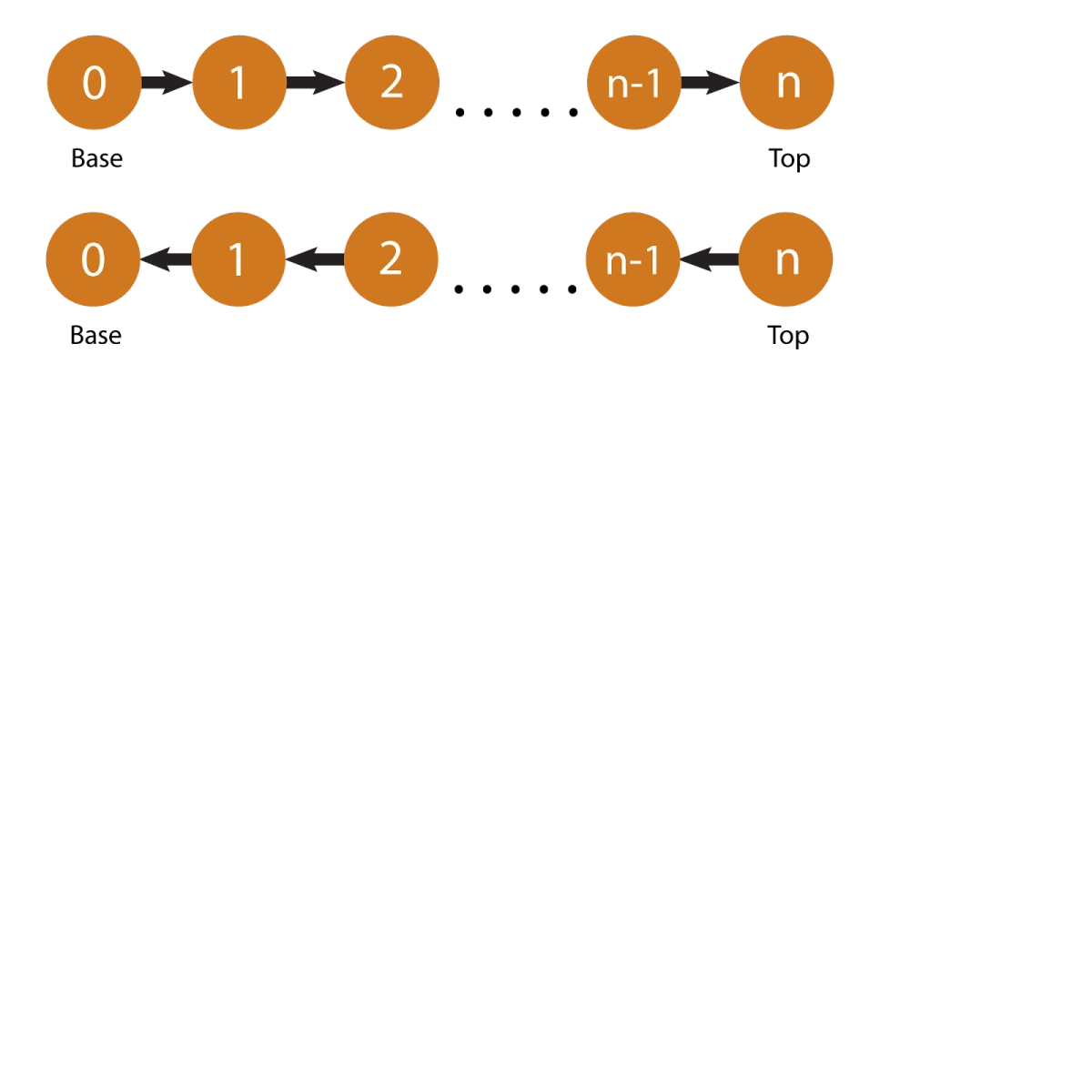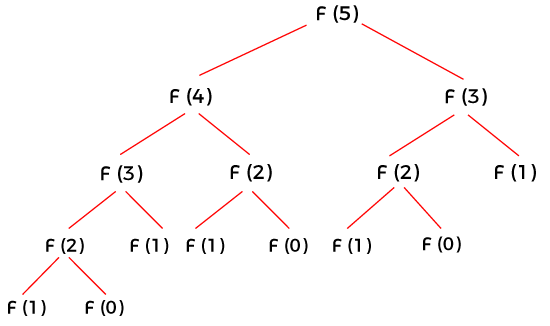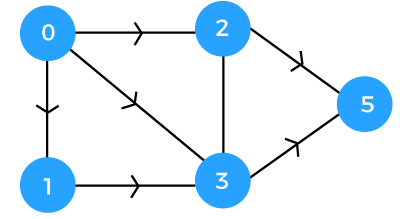New update is available. Click here to update.

# Memoization vs Tabulation

## Introduction

Dynamic programming is used to solve recursive problems iteratively and is applicable for overlapping subproblems. Problems are resolved by breaking them down into smaller subproblems and caching the overlapping subproblems to be reused to save time later. DP is usually implemented through tabulation but can also be done using memoization.

In this blog, we have also used an example and its code snippet for tabulation and memoization techniques to understand the concept better.

## What is memoization (Top-down Dynamic Programming)?

Memoization technique or top-down approach is implemented in DP algorithms where the highest-level sub-problems are solved first. Initially, it solves the highest-level subproblem and then solve the next sub-problem recursively and the next. Suppose there are two sub-problems, i.e., sub-problem A and sub-problem B. When sub-problem B is called recursively, then it can use the solution of sub-problem A, which has already been used.  Memoization saves time by avoiding solving the entire recursion tree generated by some problem with overlapping subproblems because the first problem and its sub-problems are memoized already.## What is tabulation (Bottom-Up Dynamic Programming)?

The tabulation technique or the bottom-up approach is implemented in DP algorithms where the lowest level sub-problem are solved first. In these cases, the solution to the lowest level problem helps solve the next level problem, and so on. All the sub-problems are solved iteratively in this manner till the end. Tabulation saves a lot of time when a sub-problem needs the solution from a problem that has already been solved before.## Comparison of working of Memoization and Tabulation with an example

In a Fibonacci sequence, the sum of two preceding numbers is the next number in the sequence. Eg: 0, 1, 1, 2, 3, 5, 8, 13…

The three conditions for a Fibonacci sequence are:

• If n = 0, F(n) = 0
• If n = 1, F(n)=1
• If n > 1, F(n) = F(n - 1) + f(n - 2)

Tabulation or bottom-up approach:

To find f(5) in the series 0,1,1,2,3,5...., a total of 15 calls will be needed.Source:Javatpoint

Memoization or top-down approach:

To go from the source ‘0’ to destination ‘5’, it can either be navigated through vertex ‘2’ or ‘3’. Assuming the distance between vertices ‘0’ and ‘2’ is 1 and the distance between ‘0’ and ‘3’ is 2, the decision will be made depending on the minimum distance possible.

Considering vertex ‘2’, the distance between 0 and 5 will be 1 + 1 = 2;

Considering vertex ‘3’, the distance between 0 and 5 will be 2 + 1 = 3

Since the minimum possible distance is ‘2’, we will consider vertex 2 for the operation. This will reduce the time complexity of the operation.Source:Javatpoint

Recursive code with no-cache, for a Fibonacci problem:

``````public class fibRecursive
{
int fibonacci(int i)
{
if (i < 2)
return i;
return fibonacci(i-1) + fibonacci(i-2);
}

public static void main(String[] args)
{
System.out.println(new fibRecursive().fibonacci(10));
}
}``````

## Memoization Algorithm

• This technique will require a function for cache, which is named ‘fibMemoization’ in the given snippet.
• Next, for the Fibonacci series, a function named ‘fibonacci’ is created where a number is taken as input and checked for 0 or 1, in which cases cache[n]=n. For other cases, cache[n] will be calculated by calling the function within itself, i.e., cache[n] = fibonacci( n - 1 ) + fibonacci( n - 2)
• cache[n] is returned, and the main function is written to print the output.

### Code snippet for memoization:

``````public class fibMemoization
{
int[] cache;

fibMemoization(int[] cache)
{
this.cache = cache;
}

int fibonacci(int n)
{
if (cache[n] == 0)
{
if (n < 2)
cache[n] = n;
else
cache[n] = fibonacci(n-1) + fibonacci( n-2);
}

return cache[n];
}

public static void main(String[] args)
{
int n = 10;
System.out.println(new fibMemoization(new int[n+1]).fibonacci(n));
}
}``````

### Time Complexity

The cache is checked to see if there’s already an answer stored in the nth spot and is returned if there. Otherwise, the sum of fibonacci(N - 1) and fibonacci(N - 2) is called recursively, and the sum is set to a variable. This sum is placed in the cache array at the ‘N’th spot, and then the value of the sum is returned. With this solution in memoization, whenever fibonacci(N) is called, and ‘N’ has already been solved once, cache[n] will already hold the answer and return it. The time complexity from recursively calling fibonacci(N - 1) + fibonacci(N - 2) is O(2 ^ N) and becomes a lot better with memoization - O(N).

### Space Complexity

Using the memoization technique, each ‘fibonacci’ value will be calculated only once. So, the space complexity will be O(N), where ‘N’ is the input number to ‘fibonacci’ (the array for memoization will hold ‘N’ numbers).

## Tabulation Algorithm

• An integer function is created to take input ‘N’ and create the array for the cache.
• The cache array is initialized for elements ‘0’ and ‘1’.
• Other elements of the Fibonacci series are stored in the cache using a for-loop.
• Finally, after cache[n] is returned, the main function to print output is written

### Code snippet for tabulation:

``````public class fibTabular
{
int fibonacci(int n)
{
int[] cache = new int[n+1];
cache = 0;
cache = 1;

for (int i = 2; i <= n; i++)
{
cache[i] = cache[i-1] + cache[i-2];
}

return cache[n];
}

public static void main(String[] args)
{
System.out.println(new FibonacciTabular().fibonacci(10));
}
}``````

### Time Complexity

The loop is run as long as ‘i’ is less than or equal to ‘N’ to reach the ‘N’th value correctly. The loop is iterated, and ‘i’ is incremented each time instead of recursively breaking down. This ensures that no value is recomputed and the current value is returned in O(N) time.

### Space Complexity

Since tabulation or the bottoms-up approach used constant space, the space complexity for this algorithm would be O(1).

1. What are DP problems?

Dynamic programming technique solves problems by recursively breaking them into simpler sub-problems that overlap and follow an optimal substructure. DP can solve various problems such as subset-sum, coin change, and knapsack. It can also be applied to trees.

2. Is tabulation always better than memoization?

If all subproblems need to be solved in the given problem, tabulation mostly outperforms memoization since it has no overhead for recursion. The tabulation technique can use a preallocated array instead of a hash map.

3. What is the disadvantage of memoization?

Some of the disadvantages of memoization are space overhead, slow initial execution, and extra burden on programmers as they might need to modify the code.

4. How do table entries work differently in tabulation and memoization?

Entries are filled one by one in a tabulated version, while entries are filled on-demand in the memoized version.

5. Why is it called memoization and not memorization?

Memoization is a term derived from “memorandum” in Latin, meaning “to be remembered”. It is usually truncated as “memo” and carries the meaning of turning a function into something to be remembered.

## Key takeaways

In this article, we learned the difference between the tabulation and memoization techniques and how differently they are implemented, along with examples.